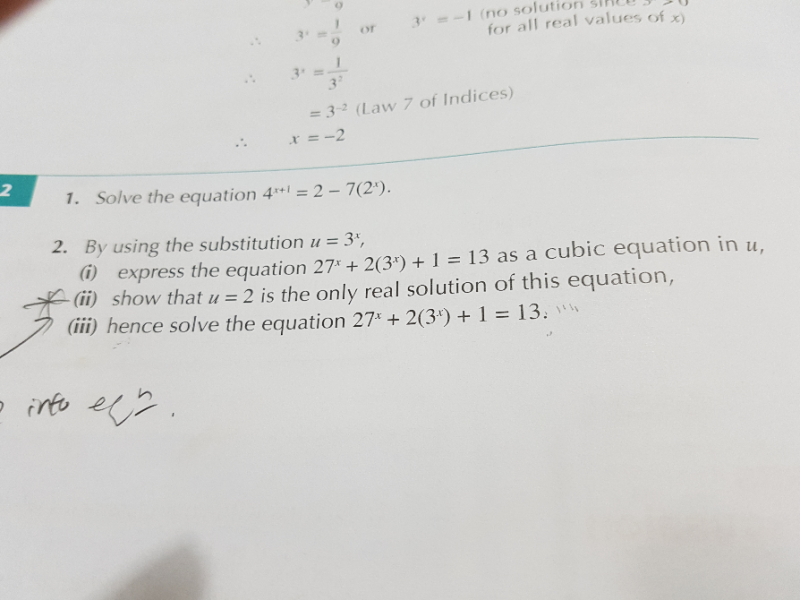QuestionPls advise on q2(iii). Thank you.

This is an indices question.

In part ii, you get

f (2) = …. = 0.

In part iii,

You will the u3 …. equation to do a long division by (u – 2) to get the quadratic quotient.

(u – 2)( ……) = 0

Since this quotient has no solution,

U – 2 = 0

3^x – 2 = 0

3^x = 2

X = lg 2 / lg 3 = …

0 Replies 0 Likes ✔Accepted Answer